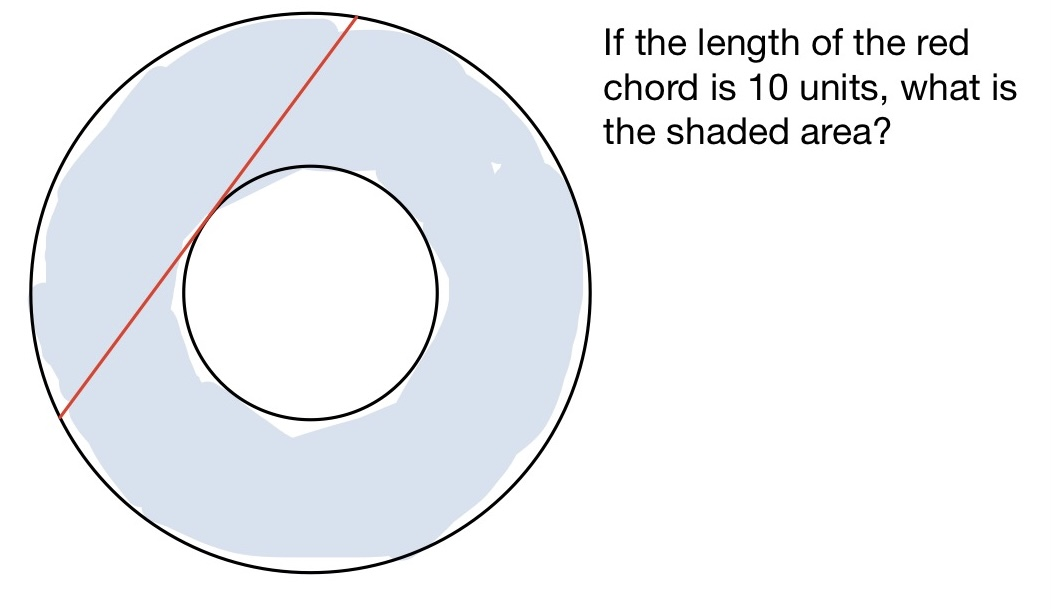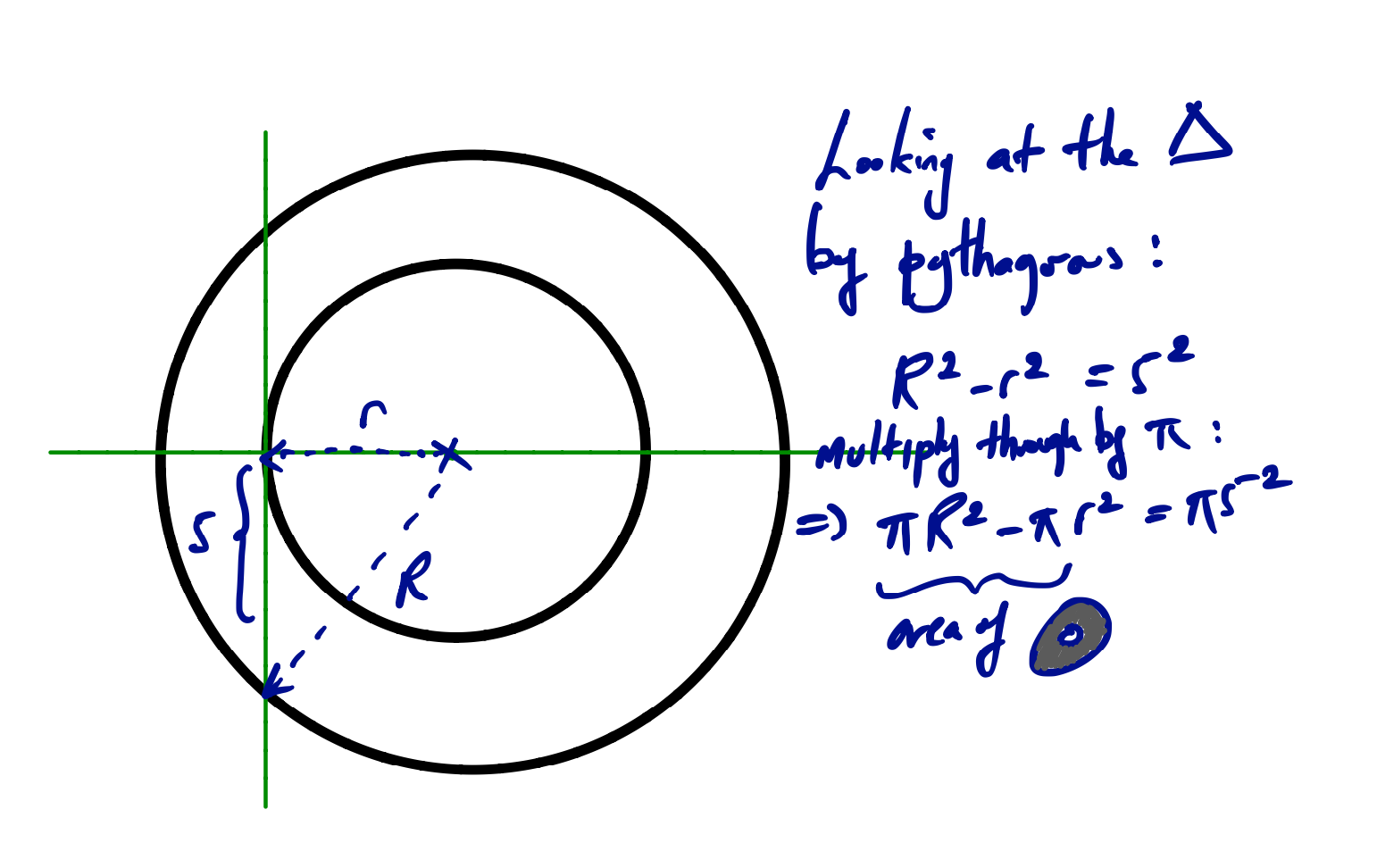## A geometric puzzle

Visible to anyone in the world

This is well-known but still surprising.Share post

25*pai?

### New comment

Correct! Can you explain the method?

### New comment

I found the following formula.

Let denote the chord as a, the radius of the large circle as R and the radius of the small circle as r. Then,

R and r are given by the equations,

R = a * square root of 3 / 2.

r = a * square root of 3 / 6.

So, the area between two circle is given by,

Area = pai * square of R - pai * square of r.

This formula is derived geometrically thinking an equilateral triangle in the big cycle, that has the chord as one of its edges.

Is this explanation right?

### New comment

Yes but we can make it even simpler! Since the radii of the two circles, call them R and r, are not known, the answer must not depend on them and we can set them to any pair of numbers that give the right chord length of 10. The simplest pair is R = 10 and r = 0, and so the shaded region is the whole of a disc of radius 5, which has radius π x 52 = 25π square units.

### New comment

Is it equal to the area of circle with radius 5? It’s a magic. I will consider well, I don’t yet understand this logic.

### New comment

My intuition told me that it was the area of a circle with radius. = half that of the chord.  It just looked right.

But I had no idea how I could prove that.

Jan

### New comment

Very interesting. I'd not seen this before.